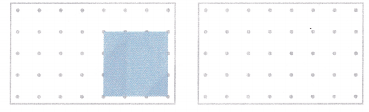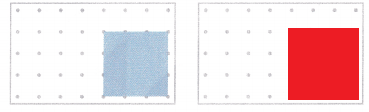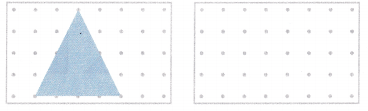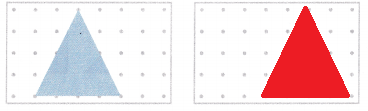# McGraw Hill Math Grade 1 Chapter 11 Lesson 6 Answer Key Drawing Shapes

All the solutions provided in McGraw Hill Math Grade 1 Answer Key PDF Chapter 11 Lesson 6 Drawing Shapes as per the latest syllabus guidelines.

## McGraw-Hill Math Grade 1 Answer Key Chapter 11 Lesson 6 Drawing Shapes

Draw

Question 1.
Look at the blue square. Draw a red square on the dots. Try to make it the same size.Explanation:
A red square is drawn with the help of blue square
both the shapes are of same sizes

Question 2.
Look at the blue triangle. Draw a red triangle on the dots. Try to make it the same size.